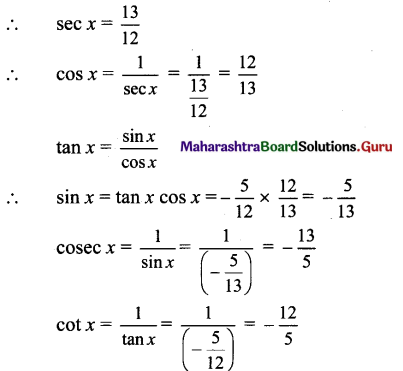# Maharashtra Board 11th Maths Solutions Chapter 2 Trigonometry – I Ex 2.1

Balbharti Maharashtra State Board Class 11 Maths Solutions Pdf Chapter 2 Trigonometry – I Ex 2.1 Questions and Answers.

## Maharashtra State Board 11th Maths Solutions Chapter 2 Trigonometry – I Ex 2.1

Question 1.
Find the trigonometric functions of 0°, 30°, 45°, 60°, 150°, 180°, 210°, 300°, 330°, – 30°, – 45°, – 60°, – 90°, – 120°, – 225°, – 240°, – 270°, – 315°
Solution:
Angle of measure 0°: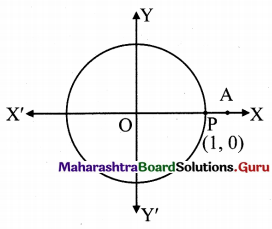Let m∠XOA = 0° = 0c
Its terminal arm (ray OA) intersects the standard
unit circle in P(1, 0).
Hence,x = 1 and y = 0
sin 0° = y = 0,
cos 0° = x = 1,
tan 0° = $$\frac{y}{x}=\frac{0}{1}$$ = 0
cot 0° = $$\frac{x}{y}=\frac{1}{0}$$ which is not defined
sec 0° = $$\frac{1}{x}=\frac{1}{1}$$ = 1
cot 0° = $$\frac{1}{y}=\frac{1}{0}$$ which is not defined,

Angle of measure 30°:
Let m∠XOA = 30°
Its terminal arm (ray OA) intersects the standard unit circle at P(x, y)
Draw seg PM perpendicular to the X-axis.
∴ ΔOMP is a 30° – 60° – 90° triangle.
OP= 1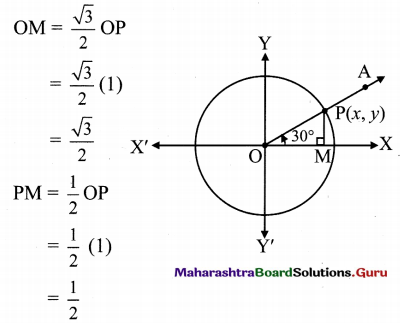Since point P lies in 1st quadrant, x > 0, y > 0
∴ x = OM = $$\frac{\sqrt{3}}{2}$$ and y = PM = $$\frac{1}{2}$$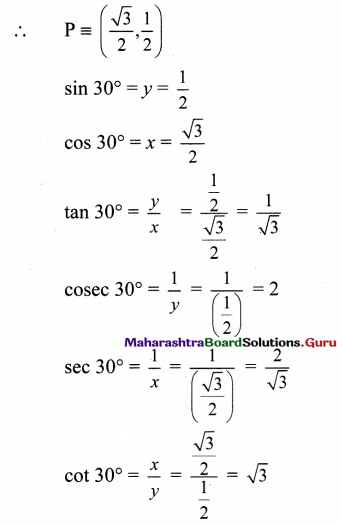Angle of measure 45°:
Let m∠XOA = 45°
Its terminal arm (ray OA) intersects the standard unit circle at P(x, y).
Draw seg PM perpendicular to the X-axis.
∴ ΔOMP is a 45° – 45° – 90° triangle.
OP = 1,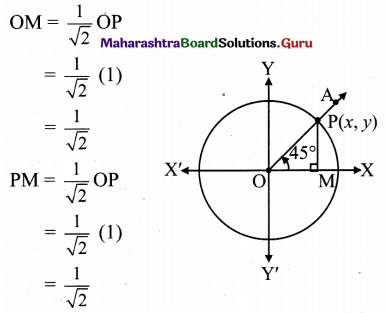Since point P lies in the 1st quadrant, x > 0, y > 0
∴ x = OM = $$\frac{1}{\sqrt{2}}$$ and
y = PM = $$\frac{1}{\sqrt{2}}$$
∴ P = ($$\frac{1}{\sqrt{2}}$$, $$\frac{1}{\sqrt{2}}$$)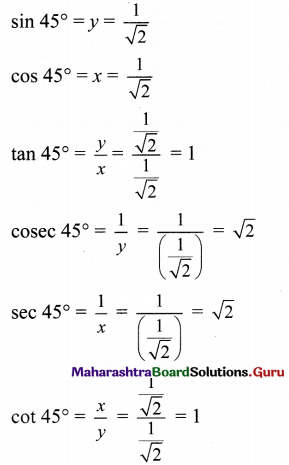Angle of measure 60°:
Let m∠XOA = 60°
Its terminal arm (ray OA) intersects the standard unit circle at P(x, y).
Draw seg PM perpendicular to the X-axis.
∴ ΔOMP is a 30° – 60° – 90° triangle.
OP= 1,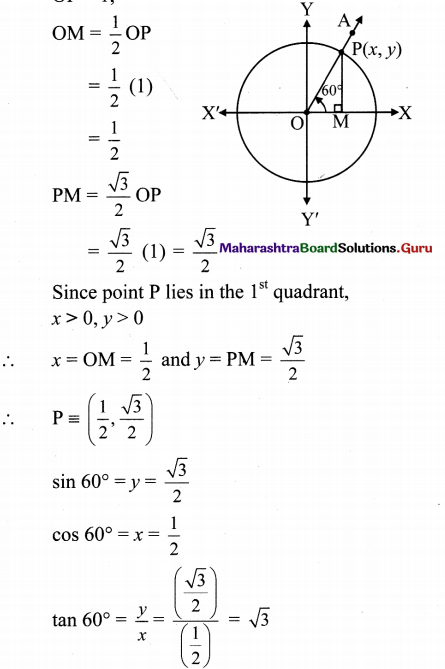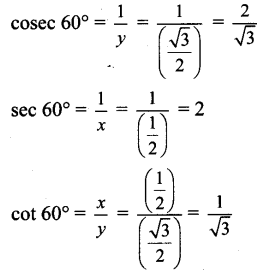Angle of measure 150°:
Let m∠XOA = 150°
Its terminal arm (ray OA) intersects the standard unit circle at P(x, y).
Draw seg PM perpendicular to the X-axis.
∴ ΔOMP is a 30° – 60° – 90° triangle.
OP= 1,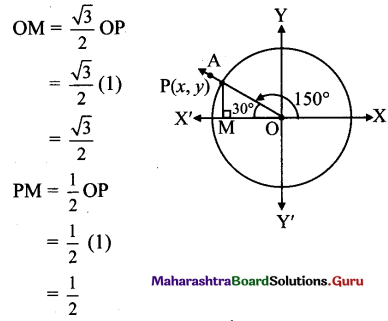Since point P lies in the 2nd quadrant, x < 0, y > 0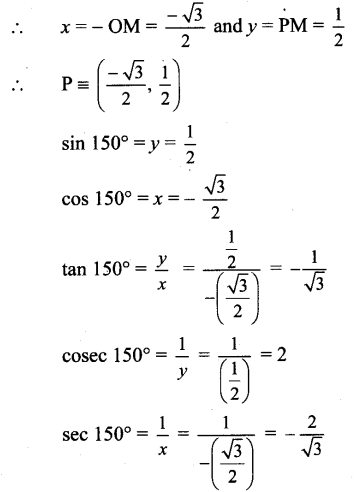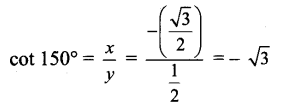Angle of measure 180°:
Let m∠XOA = 180°
Its terminal arm (ray OA) intersects the standard unit circle at P(-1, 0).
∴ x = – 1 and y = 0
sin 180° =y = 0
cos 180° = x = -1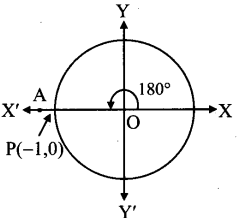tan 180° = $$\frac{y}{x}$$
= $$\frac{0}{-1}$$ = 0
Cosec 180° = $$\frac{1}{y}$$
= $$\frac{1}{0}$$
which is not defined.
sec 180°= $$\frac{1}{x}=\frac{1}{-1}$$ = -1
cot 180° = $$\frac{x}{y}=\frac{-1}{0}$$ , which is not defined.

Angle of measure 210°:
Let m∠XOA = 210°
Its terminal arm (ray OA) intersects the standard unit circle at P(x, y).
Draw seg PM perpendicular to the X-axis.
∴ ΔOMP is a 30° – 60° – 90° triangle.
OP= 1,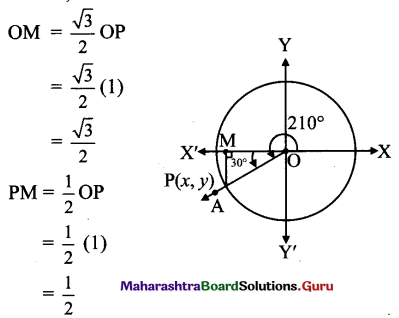Since point P lies in the 3rd quadrant, x < 0,y < 0
∴ x = -OM = $$\frac{-\sqrt{3}}{2}$$ and y = -PM = $$\frac{-1}{2}$$
∴ P ≡( $$\frac{-\sqrt{3}}{2}, \frac{-1}{2}$$ )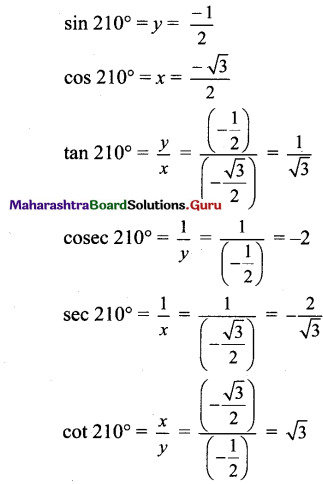Angle of measure 300°:
Let m∠XOA = 300°
Its terminal arm (ray OA) intersects the standard unit circle at P(x, y).
Draw seg PM perpendicular to the X-axis.
∴ ΔOMP is a 30° – 60° – 90° triangle.
OP = 1,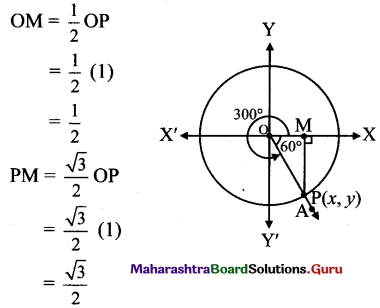Since point P lies in the 1st quadrant, x > 0,y > 0
x = OM = $$\frac{1}{2}$$ = and y = -PM = $$\frac{-\sqrt{3}}{2}$$
sin 300° = y = $$\frac{-\sqrt{3}}{2}$$
cos 300° = x = $$\frac{1}{2}$$
tan 300° = $$\frac{y}{x}=\frac{-\frac{\sqrt{3}}{2}}{\frac{1}{2}}=-\sqrt{3}$$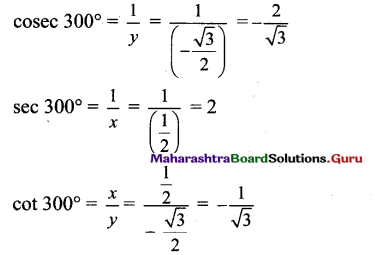Angle of measure 330°:
Let m∠XOA = 330°
Its terminal arm (ray OA) intersects the standard unit circle at P(x, y).
Draw seg PM perpendicular to the X-axis.
∴ ΔOMP is a 30° – 60° – 90° triangle.
OP= 1,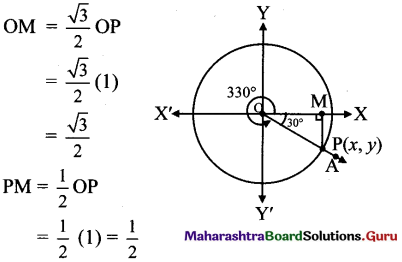Since point P lies in the 4th quadrant, x > 0, y < 0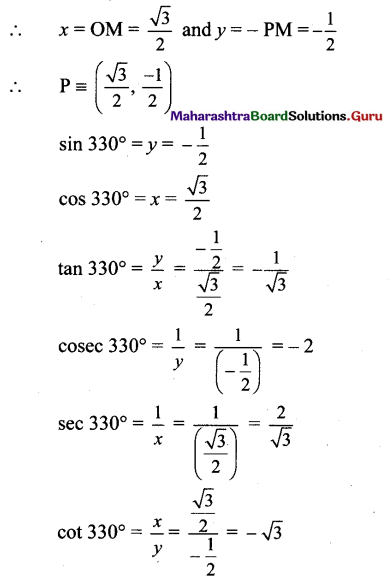Angle of measure 30°
Let m∠XOA = -30°
Its terminal arm (ray OA) intersects the standard unit circle at P(x,y).
Draw seg PM perpendicular to the X-axis.
∴ ΔOMP is a 30° – 60 — 90° triangle.
op = 1,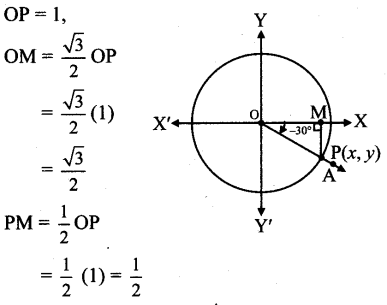Since point P lies in the 4th quadrant x > 0, y < 0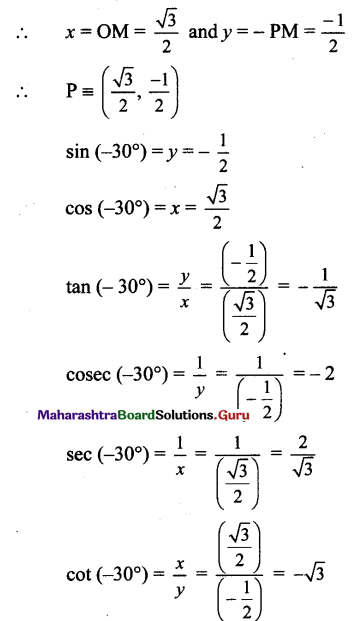Angle of measure 45°:
Let m∠XOA = 45°
Its terminal arm (ray OA) intersects the standard unit circle at P(x, y).
Draw seg PM perpendicular to the X-axis.
∴ ΔOMP is a 45° – 45° – 90° triangle.
OP = 1,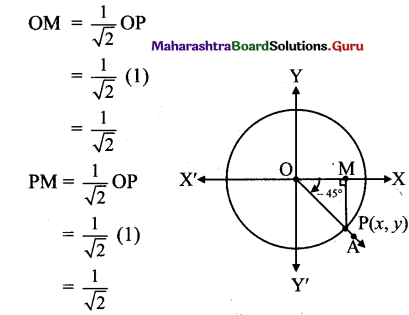Since point P lies in the 4th quadrant x > 0, y < 0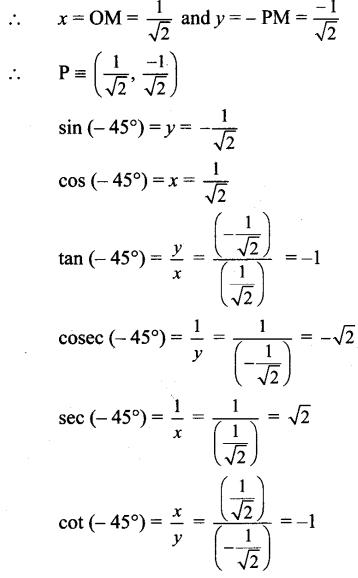[Note : Answer given in the textbook of sin (45°) = – 1/2. However, as per our calculation it is $$-\frac{1}{\sqrt{2}}$$ ]

Angle of measure (-60°):
Let m∠XOA = -60°
Its terminal arm (ray OA) intersects the standard
unit circle at P(x, y).
Draw seg PM perpendicular to the X-axis.
ΔOMP is a 30° – 60° – 90° triangle.
OP = 1,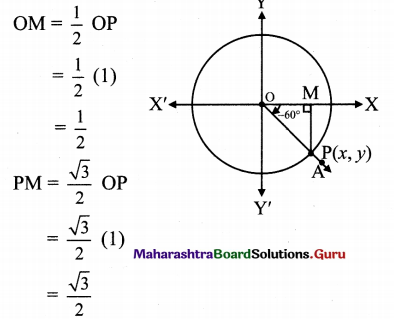Since point P lies in the 4’ quadrant,
x > 0, y < 0
x = OM =$$\frac{1}{2}$$ and y = -PM = $$-\frac{\sqrt{3}}{2}$$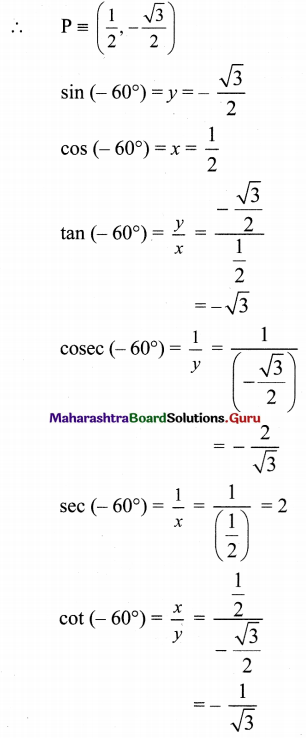Angle of measure (-90°):
Let m∠XOA = -90°
It terminal arm (ray OA) intersects the standard unit circle at P(0, -1)
∴ x = 0 and y = -1
sin (-90°) = y = -1
cos (-90°) = s = 0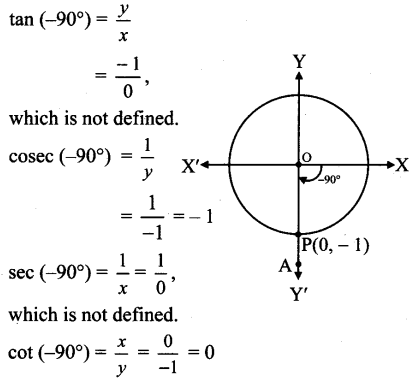Angle of measure (-120°):
Let m∠XOA = – 120°
Its terminal arm (ray OA) intersects the standard unit circle at P(x, y).
Draw seg PM perpendicular to the X-axis.
∴ ΔOMP is a 30° – 60° – 90° triangle.
OP = 1,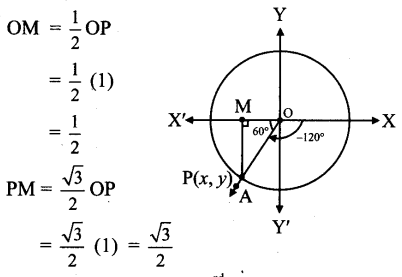Since point P lies in the 3rd quadrant, x < 0, y < 0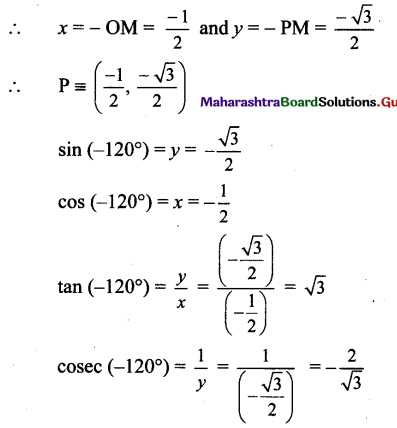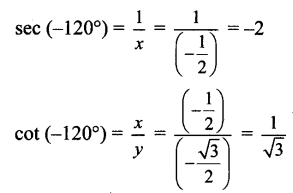Angle of measure (- 225°):
Let m∠XOA = – 225°
Its terminal arm (ray OA) intersects the standard unit circle at P(x, y).
Draw seg PM perpendicular to the X-axis.
ΔOMP is a 45° – 45° – 90° triangle.
OP = 1,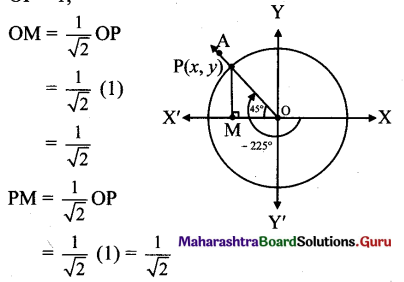Since point P lies in the 2nd quadrant, x < 0, y > 0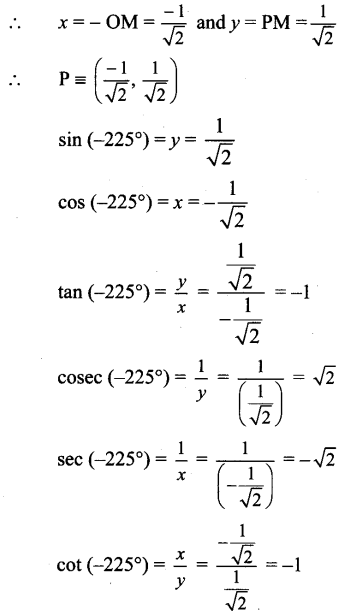Angle of measure 2400):
Let m∠XOA = 240°
Its terminal arm (ray OA) intersects the standard
unit circle at P(x, y).
Draw seg PM perpendicular to the X-axis.
∴ ΔOMP is a 30°  – 60° –  900 triangle.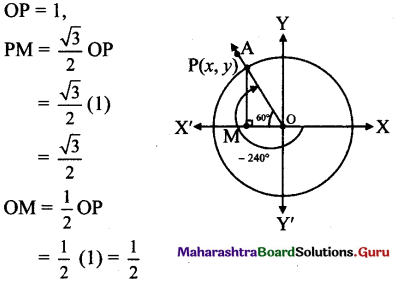Since point P lies in the 2nd quadrant, x<0, y>0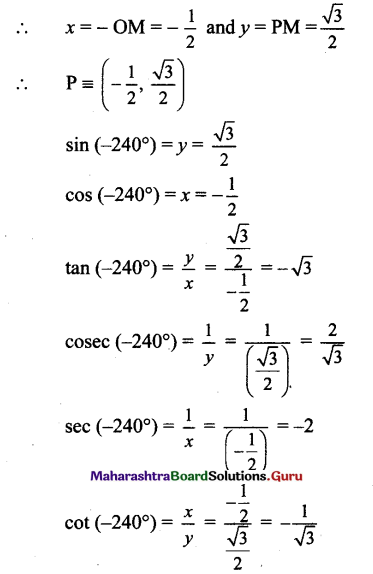Angle of measure (- 270°):
Let m∠XOA = – 270°
Its terminal arm (ray OA)
intersects the standard unit,
circle at P(0, 1).
∴ x = 0 and y = 1
sin (- 270°) = y = 1
cos (- 270°) = x = 0
tan(-270°)= $$\frac{y}{x}=\frac{1}{0}$$
which is not defined.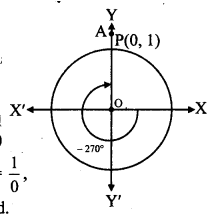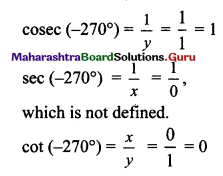Angle of measure ( 315°):
Let m∠XOA 315°
Its terminal arm (ray OA) intersects the standard unit circle at P(x,y).
Draw seg PM perpendicular to the X-axis.
ΔOMP is a 45° – 45° – 90° triangle.
OP = 1,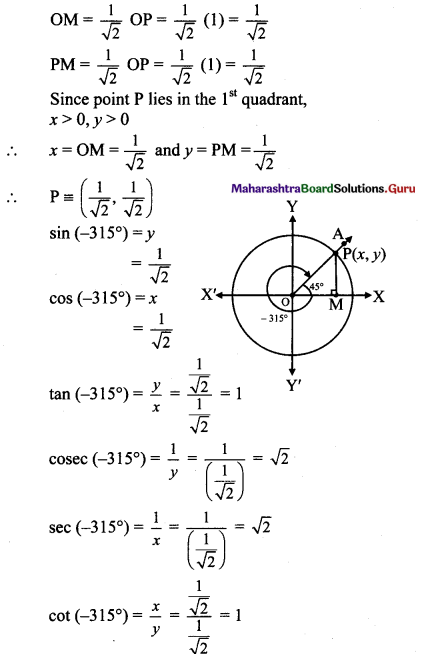Question 2.
State the signs of:
i. tan 380°
ii. cot 230°
iii 468°
Solution:
1. 380° = 360° + 20°
∴ 380° and 20° are co-terminal angles.
Since 0° < 20° <90°0,
20° lies in the l quadrant.
∴ 380° lies in the 1st quadrant,
∴ tan 380° is positive.

ii. Since, 180° <230° <270°
∴ 230° lies in the 3rd quadrant.
∴ cot 230° is positive.

iii. 468° = 360°+108°
∴ 468° and 108° are co-terminal angles.
Since 90° < 108° < 180°,
108° lies in the 2nd quadrant.
∴ 468° lies in the 2nd quadrant.
∴ sec 468° is negative.

Question 3.
State the signs of cos 4c and cos 4°. Which of these two functions is greater?
Solution:
Since 0° < 4° < 90°, 4° lies in the first quadrant. ∴ cos4° >0 …(i)
Since 1c = 57° nearly,
180° < 4c < 270°
∴ 4c lies in the third quadrant.
∴ cos 4c < 0 ………(ii)
From (i) and (ii),
cos 4° is greater.

Question 4.
State the quadrant in which 6 lies if
i. sin θ < 0 and tan θ > 0
ii. cos θ < 0 and tan θ > 0
Solution:
i. sin θ < 0 sin θ is negative in 3rd and 4th quadrants, tan 0 > 0
tan θ is positive in 1st and 3rd quadrants.
∴ θ lies in the 3rd quadrant.

ii. cos θ < 0 cos θ is negative in 2nd and 3rd quadrants, tan 0 > 0
tan θ is positive in 1st and 3rd quadrants.
∴ θ lies in the 3rd quadrant.Question 5.
Evaluate each of the following:
i. sin 30° + cos 45° + tan 180°
ii. cosec 45° + cot 45° + tan 0°
iii. sin 30° x cos 45° x lies tan 360°
Solution:
i. We know that,
sin30° = 1/2, cos 45° = $$\frac{1}{\sqrt{2}}$$ =, tan 180° = 0
sin30° + cos 45° +tan 180°
= $$\frac{1}{2}+\frac{1}{\sqrt{2}}+0=\frac{\sqrt{2}+1}{2}$$

ii. We know that,
cosec 45° = $$\sqrt{2}$$ , cot 45° = 1, tan 0° = 0
cosec 45° + cot 45° + tan 0°
= $$\sqrt{2}$$ + 1 + 0 = $$\sqrt{2}$$ + 1

iii. We know that,
sin 30° = $$\frac{1}{2}$$, cos 45° = $$\frac{1}{\sqrt{2}}$$ =, tan 360° = 0
sin 30° x cos 45° x tan 360°
= $$\left(\frac{1}{2}\right)\left(\frac{1}{\sqrt{2}}\right)$$ = 0

Question 6.
Find all trigonometric functions of angle in standard position whose terminal arm passes through point (3, – 4).
Solution:
Let θ be the measure of the angle in standard position whose terminal arm passes through P(3, -4).
∴ x = 3 and y = -4
r = OP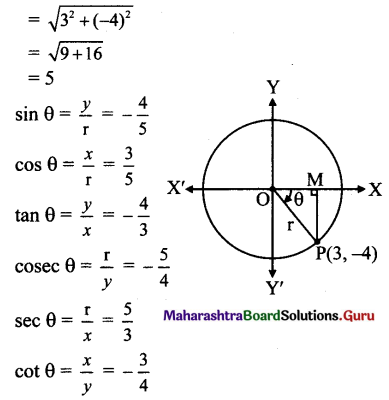Question 7.
If cos θ = $$\frac{12}{13}, 0<\theta<\frac{\pi}{2}$$ find the value of $$\frac{\sin ^{2} \theta-\cos ^{2} \theta}{2 \sin \theta \cos \theta}, \frac{1}{\tan ^{2} \theta}$$
Solution:
cos θ = $$\frac{12}{13}$$
We know that,
sin2 θ = 1 – cos2θ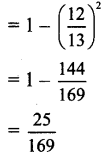∴ sin θ = ± $$\frac{5}{13}$$
Since 0 < θ < $$\frac{\pi}{2}$$ , θ lies in the 1st quadrant, ∴ sin θ > 0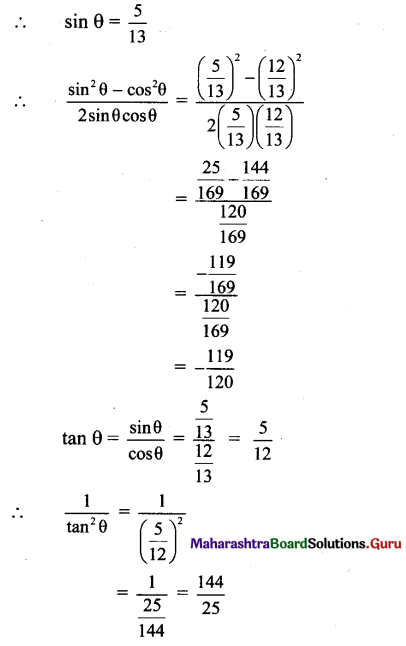Question 8.
Using tables evaluate the following:
i. 4 cot 45° – sec2 60° + sin 30°
ii.$$\cos ^{2} 0+\cos ^{2} \frac{\pi}{6}+\cos ^{2} \frac{\pi}{3}+\cos ^{2} \frac{\pi}{2}$$
Solution:
i. We know that,
cot 45° = 1, sec 60° = 2, sin 30° = 1/2
4 cot 45° – sec2 60° + sin 30°
= 4(1) – (2)2 + $$\frac{1}{2}$$
= 4 – 4 + $$\frac{1}{2}=\frac{1}{2}$$

ii. We know that,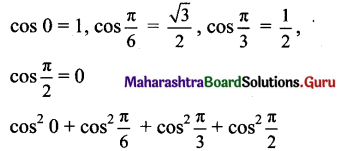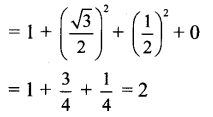Question 9.
Find the other trigonometric functions if
i. cot θ = $$-\frac{3}{5}$$, and 180 < θ < 270
ii. Sec A = $$-\frac{25}{7}$$ and A lies in the second quadrant.
iii cot x = $$\frac{3}{4}$$, x lies in the third quadrant.
iv. tan x = $$\frac{-5}{12}$$ x lies in the fourth quadrant.
Solution:
i. cot θ = $$-\frac{3}{5}$$
we know that,
sin2θ = 1 – cos2θ
= 1 – $$\left(-\frac{3}{5}\right)^{2}$$
= 1 – $$\frac{9}{25}=\frac{16}{25}$$
∴ sin θ = ± $$\frac{4}{5}$$
Since 180° < 0 < 270°,
θ lies in the 3rd quadrant.
∴ sin θ < 0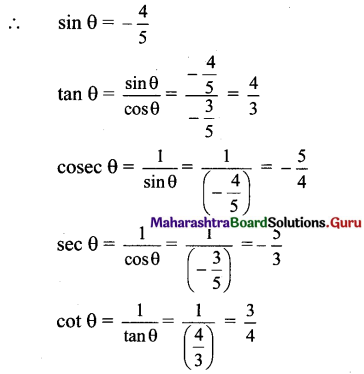Since A lies in the 2nd quadrant,
tan A < 0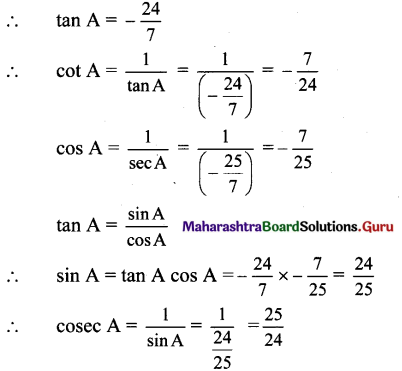iii. Given, cot x = $$\frac{3}{4}$$
We know that,
cosec2 x = 1 + cot2 x
= 1 + $$\left(\frac{3}{4}\right)^{2}=1+\frac{9}{16}=\frac{25}{16}$$
∴ cosec x = ± $$\frac{5}{4}$$
Since x lies in the 3rd quadrant, cosec x < 0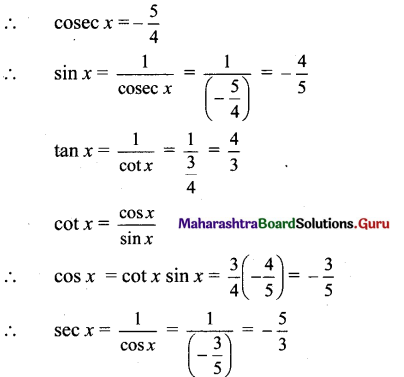iv. Given, tan x = $$-\frac{5}{12}$$
sec2 x = 1 + tan2
= 1 + $$\left(-\frac{5}{12}\right)^{2}$$
= 1 + $$\frac{25}{144}=\frac{169}{144}$$
∴ sec x = ± $$\frac{13}{12}$$
Since x lies in the 4th quadrant,
sec x > 0